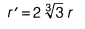# 24 solid iron spheres, each of radius r and surface area S are melted to form a sphere

24 solid iron spheres, each of radius r and surface area S are melted to form a sphere with surface area S’. Find the radius r’ of the new sphere.

Given radius of one solid iron sphere is r.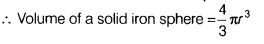.
Since, after melting, volume of new iron sphere is equal to 24 times the volume of iron sphere.
Then, volume of 24 solid iron spheres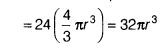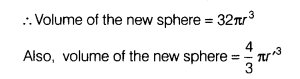According to the given condition,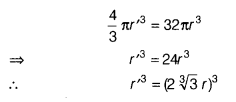On taking cube root both sides, we get r’ =2